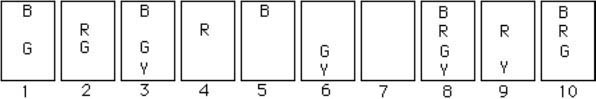# On-Sets Quiz – July 2019

 All Divisions:Depending on how it is grouped, how many cards does R – G – B equal in the Universe above? Senior Division: Double Set B – R and Symmetric Difference are both chosen for the shake. Which of these statements are false? If one player selects B – R as the Double Set and an opponent then chooses Symmetric Difference, the – in the Double Set is set subtraction, not Symmetric Difference. If one player selects Symmetric Difference and an opponent then chooses B – R as the Double Set, the – in the Double Set means Symmetric Difference. If the same player selects both Symmetric Difference and Double Set B – R, the – in B – R means set subtraction. If the same player selects both Symmetric Difference and Double Set B – R, the – in B – R means Symmetric Difference. See this and all of the other AGLOA On-Sets Quizzes here.